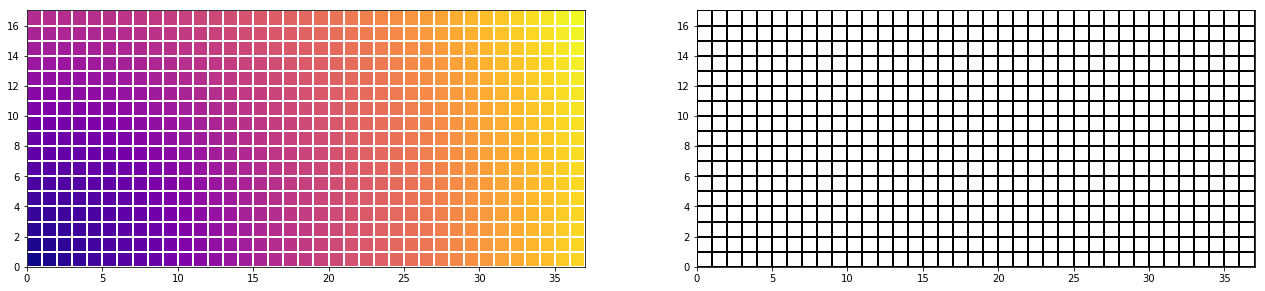.

.

.

.

.

# HealthyNumerics

HealthPoliticsEconomics | Quant Analytics | Numerics

# ComputationalFluidDynamics Articles

#### Dynamics of heat flux with an inplicite time dependent FVM-model

We model the dynamics of solar heating and of the heat ground flux. We can reconstruct why the peak of heat is in the afternoon and not at noon (when the sun is in the zenith).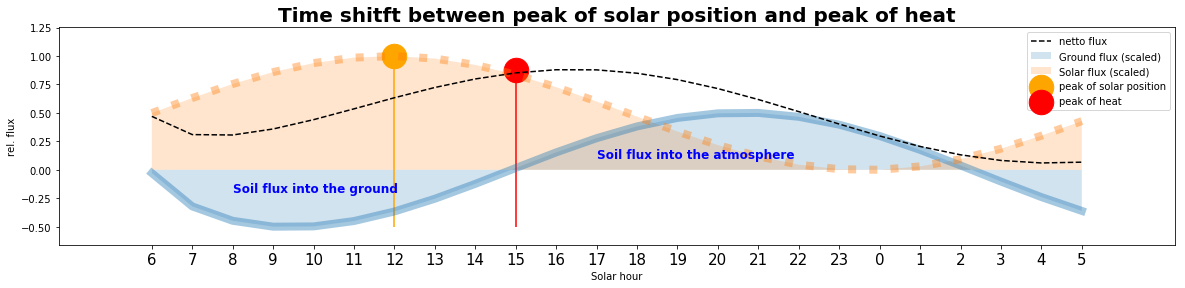#### An inplicite time dependent finite volume model (FVM) on an irregular grid for heat flow

We formulate the discretization of a heat flow model for an implicite finite volume method on an irregular grid and implement it for an 1-dimensional application. We give the detailled derivation from the partial differential equation to the FVM discretization and the implementation with vectorized Python code.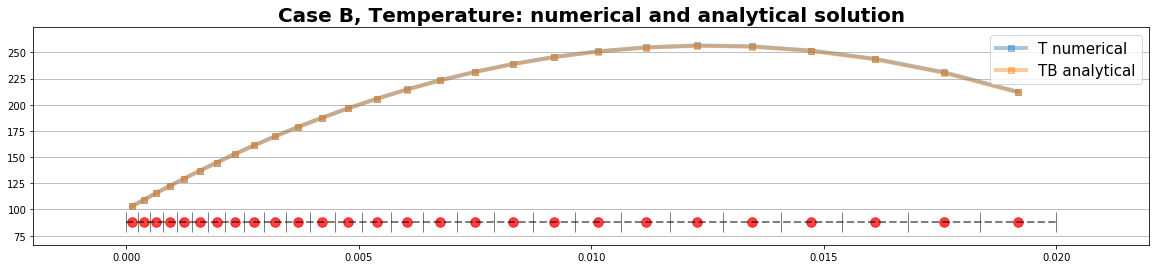#### Signal generation for distributions and heart beat (ECG wave)

We generate some basic signals and use convolution and windowing to re-construct ECG waves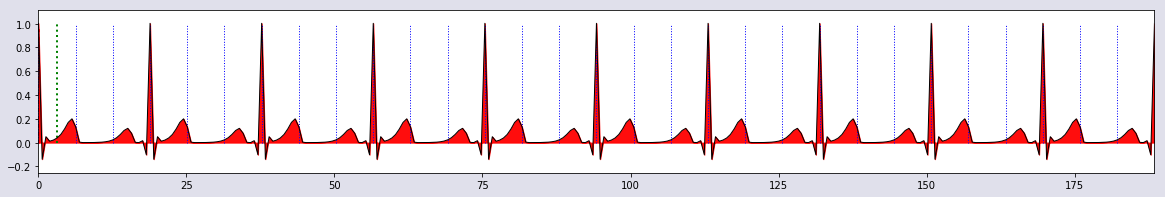#### Mapping of mean values and interpolation of distributions

We explore the building of mean values and interpolations when data have more than 1 dimension. We generate numerically a macro finite element so that arbitrary sized data can be anlalysed taking their inherent structure into account.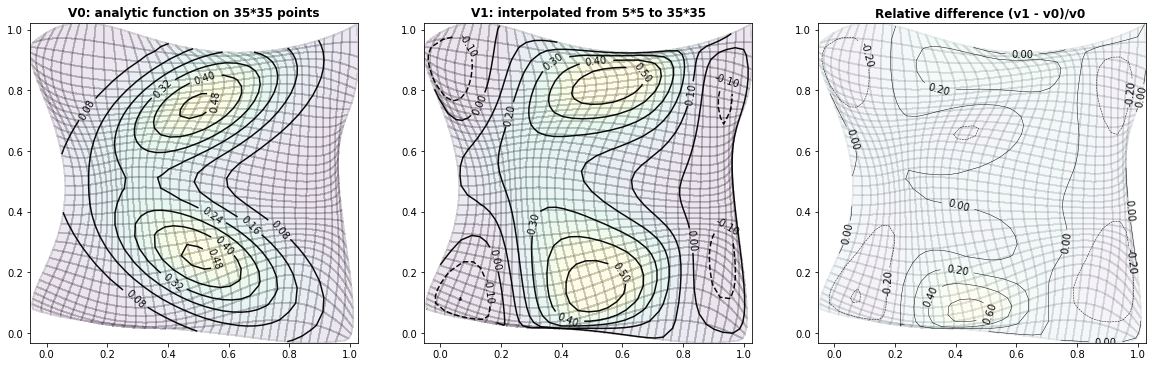#### Computational Fluid Dynamics: Markov Chain Monte Carlo in 2 dimensions

Stochastic modeling is a commonly used methodology in health economics and outcomes research (HEOR). We provide a visible insight in Markov Chain Monte Carlo modelling by a health related topic, the distribution of pollutants.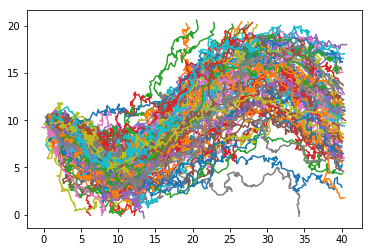#### Computational Fluid Dynamics : Estimation of the atmospheric surface fluxes

A highly parametrized schema to estimate the energy fluxes of the atmospheric surface layer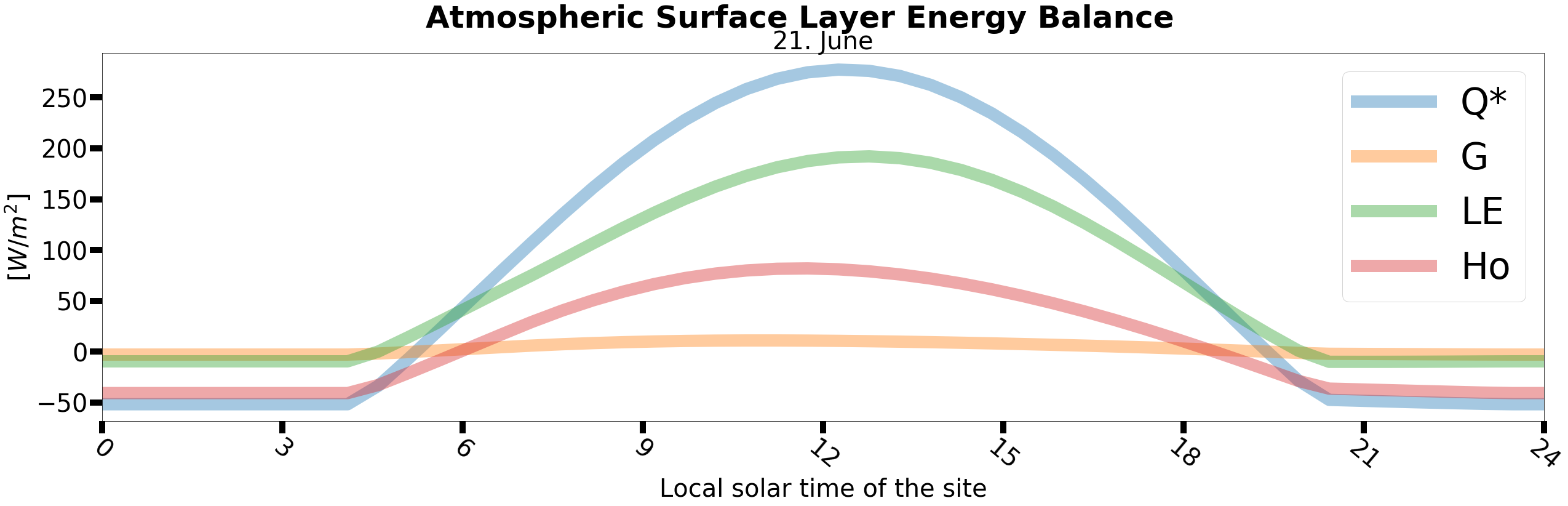#### Computational Fluid Dynamics: An explict Python Schema for the 2dim Diffusion Equation

We introduce a basic schema in computational fluid dynamics for solving the 2dimensional heat equation with a source term and constant diffusivities on an equidistant rectangular grid.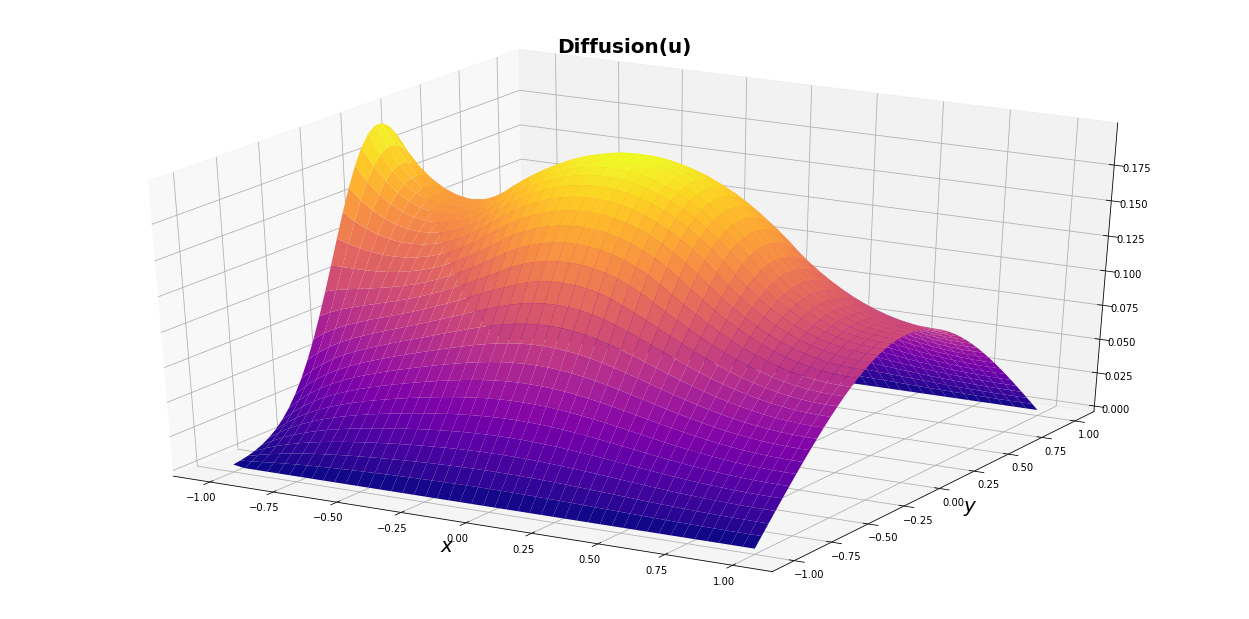#### Computational Fluid Dynamics 01: Pressure correction as mass consistent flow model

The pressure correction method is presented as Lagrange multiplier method to satisfy mass conservation#### Computational Fluid Dynamics 03: Grids for airfoils

Vectorized Python code to generate grids for airfoils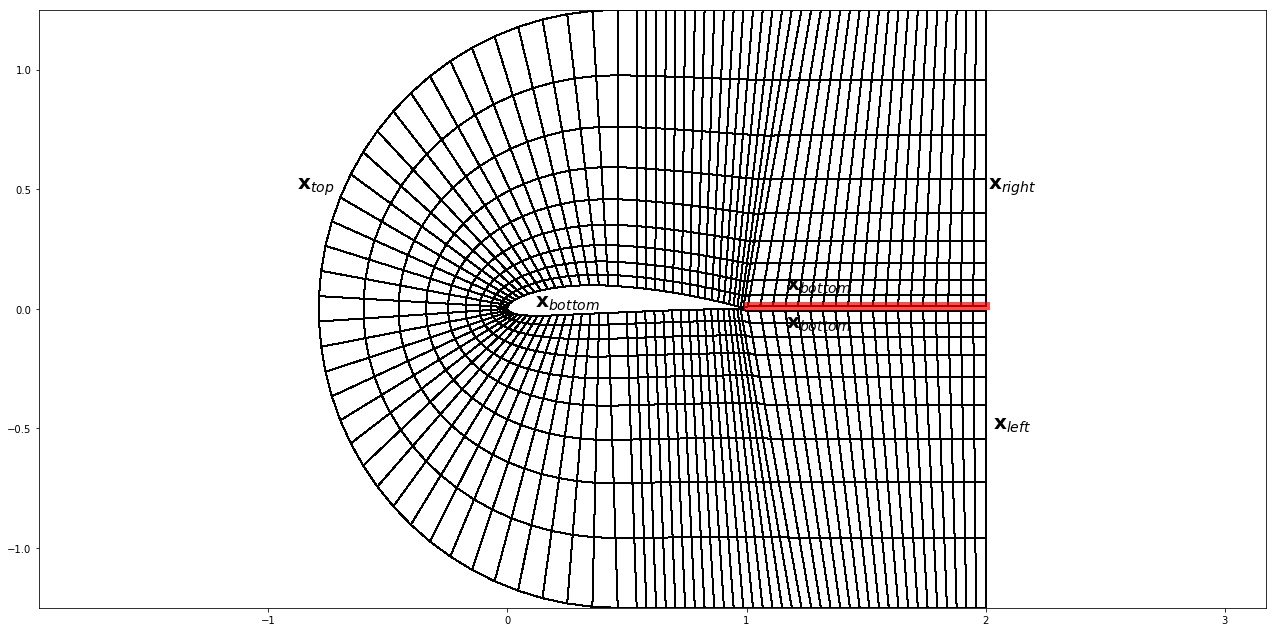#### Computational Fluid Dynamics 02: Body-fitted grids without for loops

Vectorized Python code to generate basic rectangular grids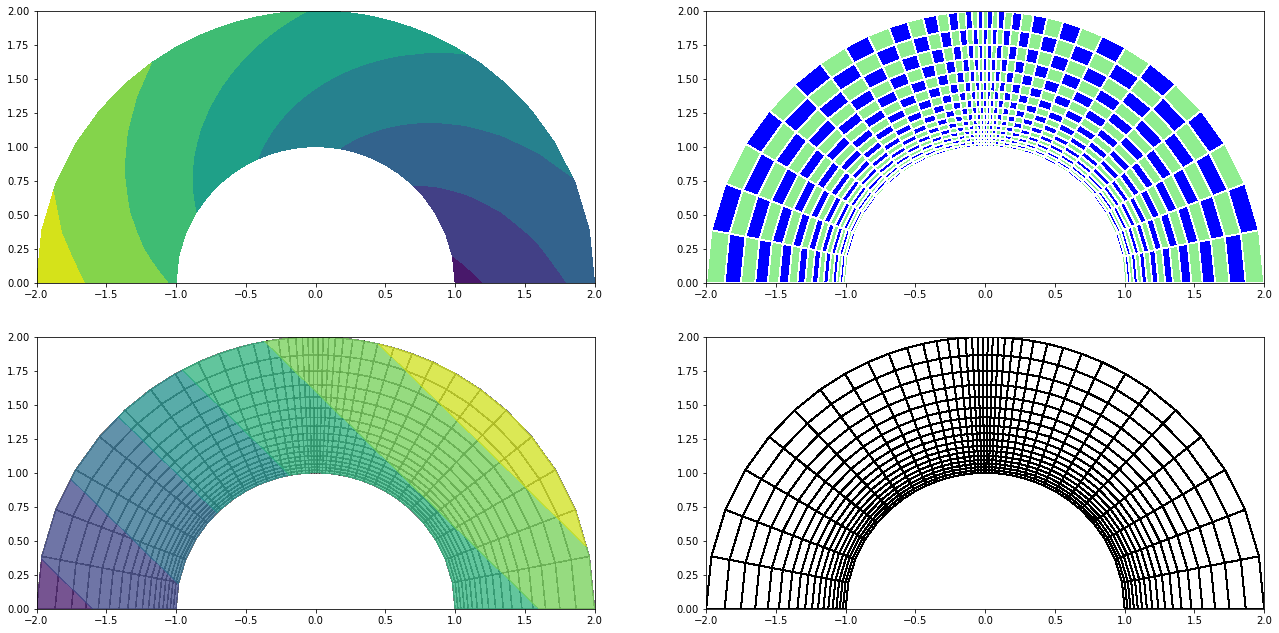#### Computational Fluid Dynamics 01: Rectangular grids without for loops

Vectorized Python code to generate basic rectangular grids